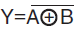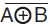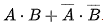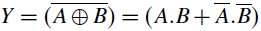# XNOR Gate | Symbol, Truth table & Circuit

A XNOR gate is a gate that gives a true (1 or HIGH) output when all of its inputs are true or when all of its inputs are false (0 or LOW). An XNOR gate is also called exclusive NOR gate or EXNOR gate. In a two input XNOR gate, the output is high (logic 1 or true) when two inputs are same.

In Boolean expression, the term XNOR is represented by symbol (⊙) and the Boolean expression is represented as Y = A ⊙ B. It is read as “A xnor B”.

It can be denoted asalso.

## XNOR gate symbol

The logic symbol of XNOR gate is shown in figure 1.

## XNOR gate truth table

The XNOR gate truth table for figure 1 is shown below.

 A B Y = A ⊙ B 0 0 1 0 1 0 1 0 0 1 1 1

From XNOR gate truth table, it can be concluded that the output will be true (logical 1 or high) when all of its inputs are true or when all of its inputs are false (0 or LOW).

Y = A ⊙ B =Y =Above algebraic expression represent the XNOR gate with inputs A and B.

### XNOR gate using OR, AND, and NOT gate

To produce XNOR gate using OR, AND, and NOT gate, the gates are joined together as shown in figure 2.

### XNOR gate using NOR gate

To produce XNOR gate using 4 NOR gates, the NOR gates are connected as shown in fig. 3.